Next: Making Life a Little Up: Commands for Math Mode Previous: Case Statements

## Diagrams

Generating diagrams is relatively straightforward. Think of the diagram as a 2-dimensional array, with each entry filled by a symbol or a blank. These arrays'' are defined in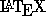as in the following example:

$$\begin{array}{rcccl} \; & \; & G &\; &\; \\ \; & \nearrow & \; & \nwarrow &\; \\ H & \; & \; & \; &\text{Stab}_G(\eta) \\ \; & \nwarrow & \; & \nearrow &\; \\ \; & \; & H_{_\rho} & \; &\; \\ \; & \; & \mid &\; &\; \\ \; & \; & K &\; &\; \end{array}$$
The argument {rcccl} works the same way as the argument to the tabular environment, described in Section 6.7. Here, {rcccl} specifies that there are 5 columns, of which the first is right-justified, the middle four centered, and the last left-justified. As in the tabular environment, each row ends with a carriage return \\, and ampersands & separate entries in a given row. Since we are making a diagram, some entries are arrows, others symbols, and many blank. The command sequence above generates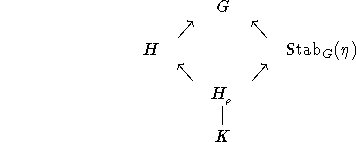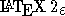includes the same automatic generator of rectangular commutative diagrams contained in A MS-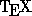when you load the amscd package.

You can then type

\begin{CD}
H^2(K_{nr}/K)   @>{\operatorname{Res}}>>   H^2(L_{nr}/L)\\
@V{\operatorname{inv}_K}VV      @VV{\operatorname{inv}_L}V \\
{\mathbb Q}/{\mathbb Z}    @>n>> {\mathbb Q}/{\mathbb Z}
\end{CD}
to make the diagram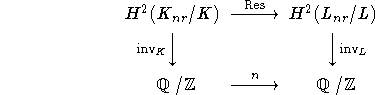Read the next section to learn how to avoid typing \operatorname all the time.

Processed by LaTeX2html